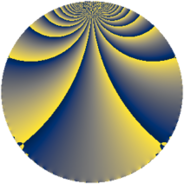Properties

 Label 1050.3.beLevel $1050$ Weight $3$ Character orbit 1050.be Rep. character $\chi_{1050}(143,\cdot)$ Character field $\Q(\zeta_{12})$ Dimension $384$ Sturm bound $720$

Related objects

Defining parameters

 Level: $$N$$ $$=$$ $$1050 = 2 \cdot 3 \cdot 5^{2} \cdot 7$$ Weight: $$k$$ $$=$$ $$3$$ Character orbit: $$[\chi]$$ $$=$$ 1050.be (of order $$12$$ and degree $$4$$) Character conductor: $$\operatorname{cond}(\chi)$$ $$=$$ $$105$$ Character field: $$\Q(\zeta_{12})$$ Sturm bound: $$720$$

Dimensions

The following table gives the dimensions of various subspaces of $$M_{3}(1050, [\chi])$$.

Total New Old
Modular forms 2016 384 1632
Cusp forms 1824 384 1440
Eisenstein series 192 0 192

Trace form

 $$384 q - 8 q^{7} + O(q^{10})$$ $$384 q - 8 q^{7} + 768 q^{16} + 32 q^{18} - 144 q^{21} - 32 q^{22} - 16 q^{28} - 60 q^{33} - 64 q^{36} + 64 q^{37} + 224 q^{42} - 64 q^{43} + 96 q^{46} + 336 q^{51} + 536 q^{57} - 112 q^{58} - 840 q^{61} + 324 q^{63} - 32 q^{67} - 64 q^{72} + 1248 q^{73} + 256 q^{78} + 256 q^{81} + 384 q^{82} + 612 q^{87} + 32 q^{88} + 968 q^{91} + 372 q^{93} + 192 q^{96} + O(q^{100})$$

Decomposition of $$S_{3}^{\mathrm{new}}(1050, [\chi])$$ into newform subspaces

The newforms in this space have not yet been added to the LMFDB.

Decomposition of $$S_{3}^{\mathrm{old}}(1050, [\chi])$$ into lower level spaces

$$S_{3}^{\mathrm{old}}(1050, [\chi]) \cong$$ $$S_{3}^{\mathrm{new}}(105, [\chi])$$$$^{\oplus 4}$$$$\oplus$$$$S_{3}^{\mathrm{new}}(210, [\chi])$$$$^{\oplus 2}$$$$\oplus$$$$S_{3}^{\mathrm{new}}(525, [\chi])$$$$^{\oplus 2}$$